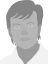# Raster Image - Convolution

The Convolution operation is an operation plug-in, which works with raster images and uses a convolution matrix to apply a simple effect on an image.

The convolution matrix is an odd sized matrix ranging from 1x1 to 31x31 items. Value of the resulting pixel is determined as a weighted average of the neighborhood pixels. The number of pixels taken into account is determined by the size of the matrix. The number in the center of the matrix belong to the actual pixel and the numbers around it determine the weights of the neighborhood pixels.

For example if a 1x5 matrix with weight (1 2 4 2 1) is used the pixels will be blurred in horizontal direction.

To simplify the specification of matrix items, a global divisor can be used keep the average values in reasonable ranges.

The bias value is added to every pixel.

## Internals

GUID: 6B506239-51B0-4416-8B1A-341C516A0F0C

 Parameter name Type Default Value Note Matrix string 0 0 00 1 00 0 0 items are separated by space, rows are separated by newline constant Div float 1.0 Bias float 0.0 added to each pixel IgnoreAlpha bool false if enabled, the convolution will be performed on premultiplied pixels and on alpha channelAnonymous